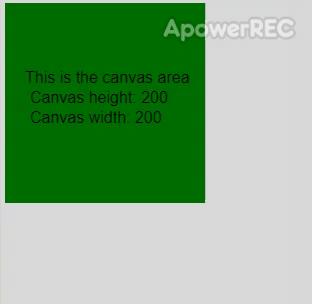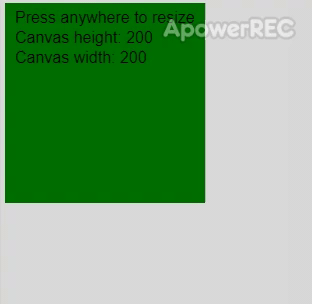GFG App
Open AppBrowser
Continue

The resizeCanvas() function is used to resize the canvas to the height and width given as parameters. The canvas is immediately redrawn after the resize takes place unless the optional ‘noRedraw’ parameter is set to true.

Syntax:

`resizeCanvas(w, h, [noRedraw])`

Parameters: This function accepts three parameters as mentioned above and described below:

• w: It is a number representing the width of the new canvas.
• h: It is a number representing the height of the new canvas.
• noRedraw: It is a boolean value which specifies if the canvas is immediately redrawn when resized.

Below example illustrates the resizeCanvas() function in p5.js:

Example 1: In this example, we fixed the increase size.

 `function` `setup() { ` `  ``createCanvas(200, 200); ` `  ``textSize(16); ` ` `  `  ``rect(0, 0, height, width); ` `  ``background(``'green'``); ` `  ``text(``"This is the canvas area"``, 20, 80); ` `  ``text(``"Canvas height: "` `+ height, 25, 100); ` `  ``text(``"Canvas width: "` `+ width, 25, 120); ` `} ` ` `  `function` `mouseClicked() { ` `  ``resizeCanvas(300, 300, ``true``); ` ` `  `  ``rect(0, 0, height, width); ` `  ``background(``'green'``); ` `  ``text(``"This is the canvas area"``, 50, 130); ` `  ``text(``"Canvas height: "` `+ height, 50, 150); ` `  ``text(``"Canvas width: "` `+ width, 50, 170); ` `} `

Output:Example 2: In this example, size depends on the click position.

 `function` `setup() { ` `  ``createCanvas(200, 200); ` `  ``textSize(16); ` ` `  `  ``rect(0, 0, height, width); ` `  ``background(``'green'``); ` `  ``text(``"Press anywhere to resize"``, 10, 20); ` `  ``text(``"Canvas height: "` `+ height, 10, 40); ` `  ``text(``"Canvas width: "` `+ width, 10, 60); ` `} ` ` `  `function` `mouseClicked(event) { ` `  ``// resize canvas to the ` `  ``resizeCanvas(event.x, event.y); ` ` `  `  ``rect(0, 0, height, width); ` `  ``background(``'green'``); ` `  ``text(``"Press anywhere to resize"``, 10, 20); ` `  ``text(``"Canvas height: "` `+ height, 10, 40); ` `  ``text(``"Canvas width: "` `+ width, 10, 60); ` `} `

Output:Online editor: https://editor.p5js.org/
Environment Setup: https://www.geeksforgeeks.org/p5-js-soundfile-object-installation-and-methods/

My Personal Notes arrow_drop_up